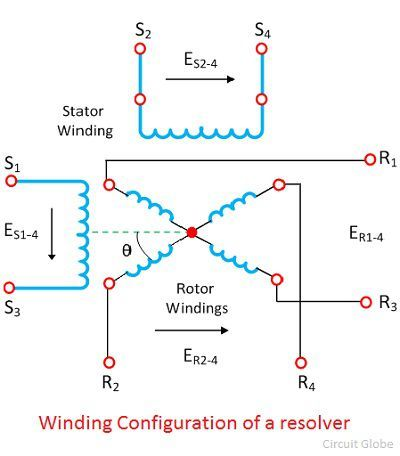# Resolver

Definition: The resolver is a rotary transformer used for conversion of the angular position of the shaft into Cartesian coordinates. It is an analogue device which has a digital counterpart. The output of the transducer has two signals, one is proportional to the sine of the angle and other is proportional to the cosine of the angle.

The resolver is a very precise electromagnetic device which consists two stators and two rotor windings. Their construction is similar to the two phase two pole wounds induction motor.

The two stator windings are placed in a same magnetic structure, but their axis is 90° apart from each other. Similarly, the rotor windings are placed in the same magnetic structure and are mutually perpendicular to each other.

The alternating voltage is applied across the stator windings which induces the alternating magnetic flux. This flux induces the voltage in the rotor windings. The output voltage of the rotor winding is directly proportional to the input voltage of the stator. The output voltage of the rotor is equal to the sine and cosine angle of the stator.

The winding configuration of the resolver is shown in the figure below.The stator winding S1 and S3 is excited by the AC source and the other winding S2 and S4 are short circuited. The output voltages are obtained from the rotor.When the stator windings are excited the output isWhen the rotor windings are excited the output is obtained from the stator winding.## Classification of Resolvers

The resolvers are classified into two groups. They are

1. Computing Resolver – Such type of resolver is used for generating the sine cosine and tangent functions. It is used for solving the geometric relationships.
2. Synchro Resolver – It is used for data transmission. They perform the functions of transmitting, receiving and as a transformer controller. It is more accurate than synchros.

### Applications of Resolvers.

The applications of resolvers are as follows

1. It is used in vector resolution, which is a process of splitting the vector into the various part.
2. It is used for determining the vector angle and component.
3. The resolver is used for controlling the amplitude of pulses and also in pulse resolution.
4. It is used for phase shifting.

The resolver can accurately convert the polar value into a rectangular form. The rotation of the shaft gives the polar value and catering coordinates convert it into rectangular form (x, y).